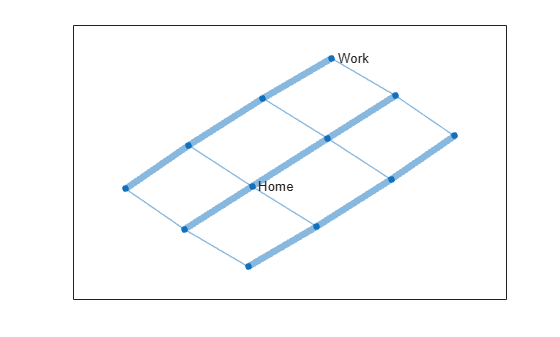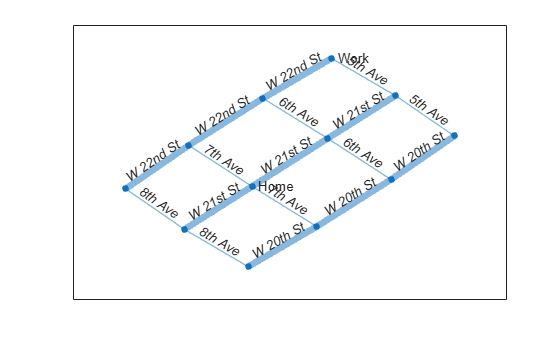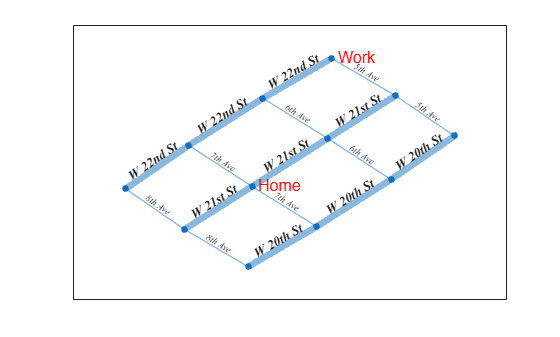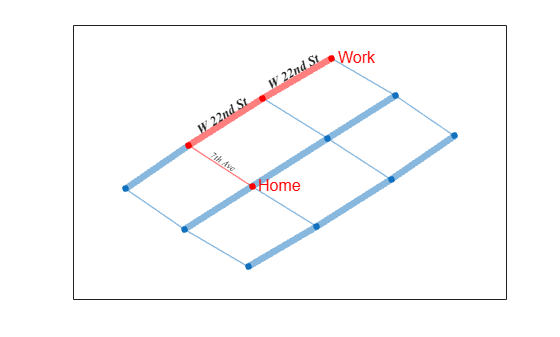# Label Graph Nodes and Edges

This example shows how to add and customize labels on graph nodes and edges.

### Create and Plot Graph

Create a graph representing the gridded streets and intersections in a city. Add weights to the edges so that the main avenues and cross streets appear differently in the plot. Plot the graph with the edge line widths proportional to the weight of the edge.

```s = [1 1 2 2 3 4 4 5 5 6 7 7 8 8 9 10 11]; t = [2 4 3 5 6 5 7 6 8 9 8 10 9 11 12 11 12]; weights = [1 5 1 5 5 1 5 1 5 5 1 5 1 5 5 1 1]; G = graph(s,t,weights); P = plot(G,'LineWidth',G.Edges.Weight);```For graphs with 100 or fewer nodes, MATLAB® automatically labels the nodes using the numeric node indices or node names (larger graphs omit these labels by default). However, you can change the node labels by adjusting the `NodeLabel` property of the `GraphPlot` object `P` or by using the `labelnode` function. Therefore, even if the nodes have names, you can use labels that are different from the names.

Remove the default numeric node labels. Label one of the intersections as `Home` and another as `Work`.

```labelnode(P,1:12,'') labelnode(P,5,'Home') labelnode(P,12,'Work')```The edges in a plotted graph are not labeled automatically. You can add edge labels by changing the value of the `EdgeLabel` property of the `GraphPlot` object `P` or by using the `labeledge` function.

Add edge labels for streets in New York City. The order of the edges is defined in the `G.Edges` table of the graph, so the order of the labels you specify must respect that order. It is convenient to store edge labels directly in the `G.Edges` table, so that the edge name lives right next to the other edge information.

`G.Edges`
```ans=17×2 table EndNodes Weight ________ ______ 1 2 1 1 4 5 2 3 1 2 5 5 3 6 5 4 5 1 4 7 5 5 6 1 5 8 5 6 9 5 7 8 1 7 10 5 8 9 1 8 11 5 9 12 5 10 11 1 ⋮ ```

This example has 17 edges but only 7 unique street names. Therefore, it makes sense to define the street names in a cell array and then index into the cell array to retrieve the desired street name for each edge. Add a variable to the `G.Edges` table containing the street names.

```streets = {'8th Ave' '7th Ave' '6th Ave' '5th Ave' ... 'W 20th St' 'W 21st St' 'W 22nd St'}'; inds = [1 5 1 6 7 2 5 2 6 7 3 5 3 6 7 4 4]; G.Edges.StreetName = streets(inds); G.Edges```
```ans=17×3 table EndNodes Weight StreetName ________ ______ _____________ 1 2 1 {'8th Ave' } 1 4 5 {'W 20th St'} 2 3 1 {'8th Ave' } 2 5 5 {'W 21st St'} 3 6 5 {'W 22nd St'} 4 5 1 {'7th Ave' } 4 7 5 {'W 20th St'} 5 6 1 {'7th Ave' } 5 8 5 {'W 21st St'} 6 9 5 {'W 22nd St'} 7 8 1 {'6th Ave' } 7 10 5 {'W 20th St'} 8 9 1 {'6th Ave' } 8 11 5 {'W 21st St'} 9 12 5 {'W 22nd St'} 10 11 1 {'5th Ave' } ⋮ ```

Update the `EdgeLabel` property to reference these street names.

`P.EdgeLabel = G.Edges.StreetName;`The node and edge labels in a graph plot have their own properties that control the appearance and style of the labels. Since the properties are decoupled, you can use different styles for the node labels and the edge labels.

For Node labels, you can adjust:

• `NodeLabel`

• `NodeLabelColor`

• `NodeFontName`

• `NodeFontSize`

• `NodeFontWeight`

• `NodeFontAngle`

For Edge labels, you can adjust:

• `EdgeLabel`

• `EdgeLabelColor`

• `EdgeFontName`

• `EdgeFontSize`

• `EdgeFontWeight`

• `EdgeFontAngle`

Use these properties to adjust the fonts in this example with New York City Streets:

• Change `NodeFontSize` and `NodeLabelColor` so that the intersection labels are 12 pt. font and red.

• Change `EdgeFontWeight`, `EdgeFontAngle`, and `EdgeFontSize` to use a larger, bold font for streets in one direction and a smaller, italic font for streets in the other direction.

• Change `EdgeFontName` to use Times New Roman for the edge labels.

You can use the `highlight` function to change the graph properties of a subset of the graph edges. Create a logical index `isAvenue` that is `true` for edge labels containing the word `'Ave'`. Using this logical vector as an input to `highlight`, label all of the Avenues in one way, and all of the non-Avenues another way.

```P.NodeFontSize = 12; P.NodeLabelColor = 'r'; isAvenue = contains(P.EdgeLabel, 'Ave'); highlight(P, 'Edges', isAvenue, 'EdgeFontAngle', 'italic', 'EdgeFontSize', 7); highlight(P, 'Edges', ~isAvenue, 'EdgeFontWeight', 'bold', 'EdgeFontSize', 10); P.EdgeFontName = 'Times New Roman';```### Highlight Edges

Find the shortest path between the Home and Work nodes and examine which streets are on the path. Highlight the nodes and edges on the path in red and remove the edge labels for all edges that are not on the path.

`[path,d,pathEdges] = shortestpath(G,5,12)`
```path = 1×4 5 6 9 12 ```
```d = 11 ```
```pathEdges = 1×3 8 10 15 ```
`G.Edges.StreetName(pathEdges,:)`
```ans = 3x1 cell {'7th Ave' } {'W 22nd St'} {'W 22nd St'} ```
```highlight(P,'Edges',pathEdges,'EdgeColor','r') highlight(P,path,'NodeColor','r') labeledge(P, setdiff(1:numedges(G), pathEdges), '')```